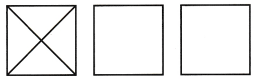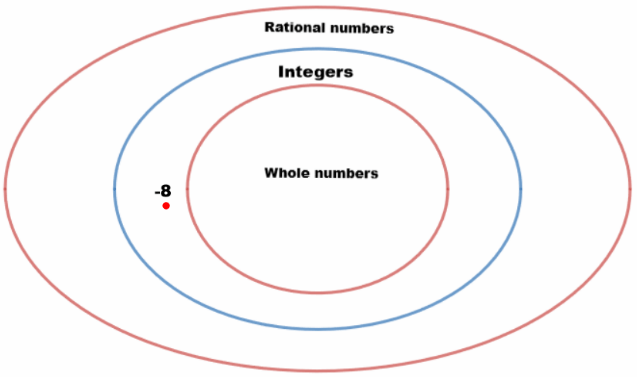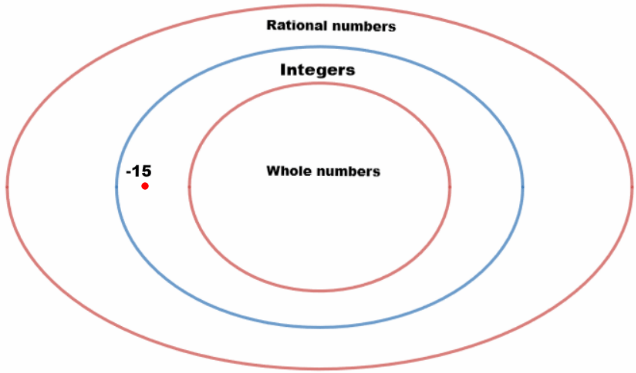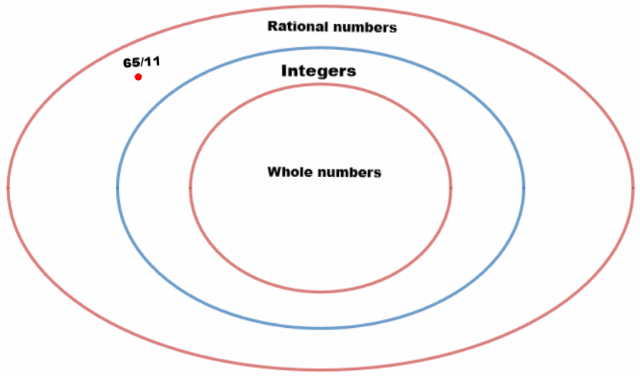# Texas Go Math Grade 6 Lesson 2.1 Answer Key Classifying Rational Numbers

Refer to our Texas Go Math Grade 6 Answer Key Pdf to score good marks in the exams. Test yourself by practicing the problems from Texas Go Math Grade 6 Lesson 2.1 Answer Key Classifying Rational Numbers.

## Texas Go Math Grade 6 Lesson 2.1 Answer Key Classifying Rational Numbers

Representing Division as a Fraction

Alicia and her friends Brittany, Kenji and Ellis are taking a pottery class. The four friends have to share 3 blocks of clay. How much clay will each of them receive if they divide the 3 blocks evenly?

(A) The top faces of the 3 blocks of clay can be represented by squares. Use the model to show the part of each block that each friend will receive. Explain.(B) Each piece of one square is equal to what fraction of a block of clay?

(C) Explain how to arrange the pieces to model the amount of clay each person gets. Sketch the model.(D) What fraction of a square does each person’s pieces cover? Explain.

(E) How much clay will each person receive?

(F) Multiple Representations How does this situation represent division?

Reflect

Question 1.
Communicate Mathematical Ideas 3 ÷ 4 can be written $$\frac{3}{4}$$ How are the dividend and divisor of a division expression related to the parts of a fraction?
Dividend and divisor are connected to the fraction as numerator and denominator respectively!

dividend – numerator;
divisor – denominator

Question 2.
Analyze Relationships How could you represent the division as a fraction if 5 people shared 2 blocks? if 6 people shared 5 blocks?
If 5 people shared 2 blocks, we can represent this as:
$$\frac{5}{2}$$

If 6 people shared 5 blocks, we can represent this as:
$$\frac{6}{5}$$

Write each rational number as $$\frac{a}{b}$$.

Question 3.
– 15 ____________
Number – 15 can be written by fraction $$\frac{a}{b}$$ as:
– $$\frac{15}{1}$$

Question 4.
0.31 ____________
Number 0.31 can be written by fraction $$\frac{a}{b}$$ as:
$$\frac{31}{100}$$

Question 5.
4$$\frac{5}{9}$$ ____________
Number 4$$\frac{5}{9}$$ can be written by fraction $$\frac{a}{b}$$ as:
$$\frac{41}{9}$$

Question 6.
62 ____________
Number 62 can be written by fraction $$\frac{a}{b}$$ as:
$$\frac{62}{1}$$

Question 7.
Analyze Relationships Name two integers that are not also whole numbers.
Here, we pick numbers which are negative so they don’t get involved in whole numbers group:
– 7, – 10

Question 8.
Analyze Relationships Describe how the Venn diagram models the relationship between rational numbers, integers, and whole numbers.
Venn diagram shows reference between groups.
It shows that Whole numbers are included in Integers and Integers in Rational numbers.

Place each number in the Venn diagram. Then classify each number by indicating in which set or sets it belongs.Question 9.
14.1 ____________Number 14.1 belongs to Rational Numbers

Question 10.
7$$\frac{1}{5}$$ ____________Please note that I wrote mixed number 7$$\frac{1}{5}$$ as 7.2 because i cannot use alignment in editing picture.
Number 7$$\frac{1}{5}$$ belongs to Rational numbers

Question 11.
– 8 ____________Number – 8 belongs to Integers.

Question 12.
101 ____________Number 101 belongs to whole numbers

Question 1.
Sarah and four friends are decorating picture frames with ribbon. They have 4 rolls of ribbon to share evenly. (Explore Activity)

a. How does this situation represent division?
This situation can be represented by division as each person gets same amount of ribbon, therefore they have to split it into equal parts!

b. How much ribbon does each person receive?
If Sarah has 4 friends, that means that 4 rolls of ribbon will be shared equally only if each gets:
$$\frac{4}{5}$$

Write each rational number in the form where a and b are integers.

Question 2.
0.7 ___________
Number 0.7 can be written in form of $$\frac{a}{b}$$ as:
$$\frac{7}{10}$$

Question 3.
– 29 ___________
Number – 29 can be written in form of $$\frac{a}{b}$$ as:
$$-\frac{29}{1}$$

Question 4.
8 ___________
Mixed number 8$$\frac{1}{3}$$ can be written in form of $$\frac{a}{b}$$ as:
$$\frac{25}{3}$$

Place each number in the Venn diagram. Then classify each number by indicating in which set or sets each number belongs.Question 5.
– 15 ___________Number – 15 belongs to set of Integers

Question 6.
5$$\frac{10}{11}$$ ___________Please note that i wrote mixed number 5$$\frac{10}{11}$$ as 65/11 since i cannot use alignment in picture editing!
Number 5$$\frac{10}{11}$$ beLongs to set of Rational numbers.

Essential Question Check-In

Question 7.
How is a rational number that is not an integer different from a rational number that is an integer?
Number that is Rational and not Integer can not be written as whole number or its opposite.

List two numbers that fit each description. Then write the numbers in the appropriate location on the Venn diagram.Question 8.
Integers that are not whole numbers
Integers that are not Whole numbers are opposites of Whole numbers: – 3, – 5Question 9.
Rational numbers that are not integers
Rational numbers that are not Integers are numbers that are not Whole numbers or their opposites: $$\frac{5}{3}, \frac{7}{8}$$Please note that i wrote $$\frac{5}{3}$$ instead of $$\frac{5}{3}$$ and $$\frac{7}{8}$$ instead of $$\frac{7}{8}$$

Question 10.
Multistep A nature club is having its weekly hike. The table shows how many pieces of fruit and bottles of water each member of the club brought to share.a. If the hikers want to share the fruit evenly, how many pieces should each person receive?
If hikers wanted to share 14 pieces of fruit evenly, each of them would get: $$\frac{14}{4}$$

b. Which hikers received more fruit than they brought on the hike?
To calculate, which of hikers got more fruit than brought, we have to change the format of our number to mixed number We know how to do it:
$$\frac{14}{4}$$ = $$\frac{4}{4}+\frac{4}{4}+\frac{4}{4}+\frac{2}{4}$$
= 1 + 1 + 1 + $$\frac{2}{4}$$
= 3 + $$\frac{2}{4}$$
= 3$$\frac{2}{4}$$
Now we can conclude that Hendrick and Baxer will get more pieces than they brought1

c. The hikers want to share their water evenly so that each member has the same amount. How much water does each hiker receive?
As there are 17 bottles of water altogether, if they split it in 4 parts that means each hiker is going to get: $$\frac{17}{4}$$ bottles.
As this is not Whole number, some hiker will have to bring that one extra bottle so they could share it between
themselves!

Question 11.
Sherman has 3 cats and 2 dogs. He wants to buy a toy for each of his pets. Sherman has $22 to spend on pet toys. How much can he spend on each pet? Write your answer as a fraction and as an amount in dollars and cents. Answer: As Sherman has 5 pets in total. That means he has to share$22 into 5 equal parts.
He will spend on each: $$$\frac{22}{5}$$ He will spend$4 and 40 cents for each pet!

Question 12.
A group of 5 friends is sharing 2 pounds of trail mix. Write a division problem and a fraction to represent this situation.
Since 5 of them share 2 pounds of milk, that means each will get:
$$\frac{5}{2}$$ pounds.

Question 13.
Vocabulary A _____________ diagram can represent set relationships visually.
A Venn diagram can visually represent set relationships.

Financial Literacy For 14-16, use the table. The table shows Jason’s utility bills for one month. Write a fraction to represent the division in each situation. Then classify each result by indicating the set or sets to which it belongs.Question 14.
Jason and his 3 roommates share the cost of the electric bill evenly.
So, electricity payments bill splits into 4 equal parts!
We can express it via fraction as: $$$\frac{108}{4}$$ This number belongs to set of whole numbers as its equivalent to number: 27 Question 15. Jason plans to pay the water bill with 2 equal payments. Answer: Jason is paying water bill with 2 equal payments. That means he is going to pay:$$$\frac{35}{2}$$
This number belongs to set of Rational numbers.

Question 16.
Jason owes $15 for last month’s gas bill also. The total amount of the two gas bills is split evenly among the 4 roommates. Answer: As he owes$15 from last month, they will have to pay: $15 +$14 = $29 They split it into 4 parts and each pays:$$$\frac{29}{4}$$
This number belongs to set of Rational numbers.

Question 17.
Lynn has a watering can that holds 16 cups of water, and she fills it half full. Then she waters her 15 plants so that each plant gets the same amount of water. How many cups of water will each plant get?
Glass can holds 16 cups of water, and she filled it half, so she filled it with 8 cups of water.
Now, we know that if she has 15 plants, and wants to split that water equally,
she will spend: $$\frac{8}{15}$$
This number belongs to set of Rational numbers.

H.O.T. Focus on Higher Order Thinking

Question 18.
Critique Reasoning DaMarcus says the number $$\frac{24}{6}$$ belongs only to the set of rational numbers. Explain his error.
Number $$\frac{24}{6}$$ can be written without fraction as 4.
Therefore, it belongs to set of Integers and Whole numbers also.

Question 19.
Analyze Relationships Explain how the Venn diagrams in this lesson show that all integers and all whole numbers are rational numbers.